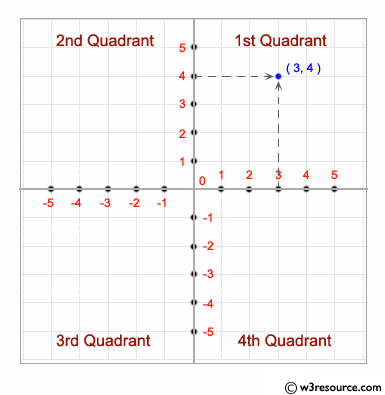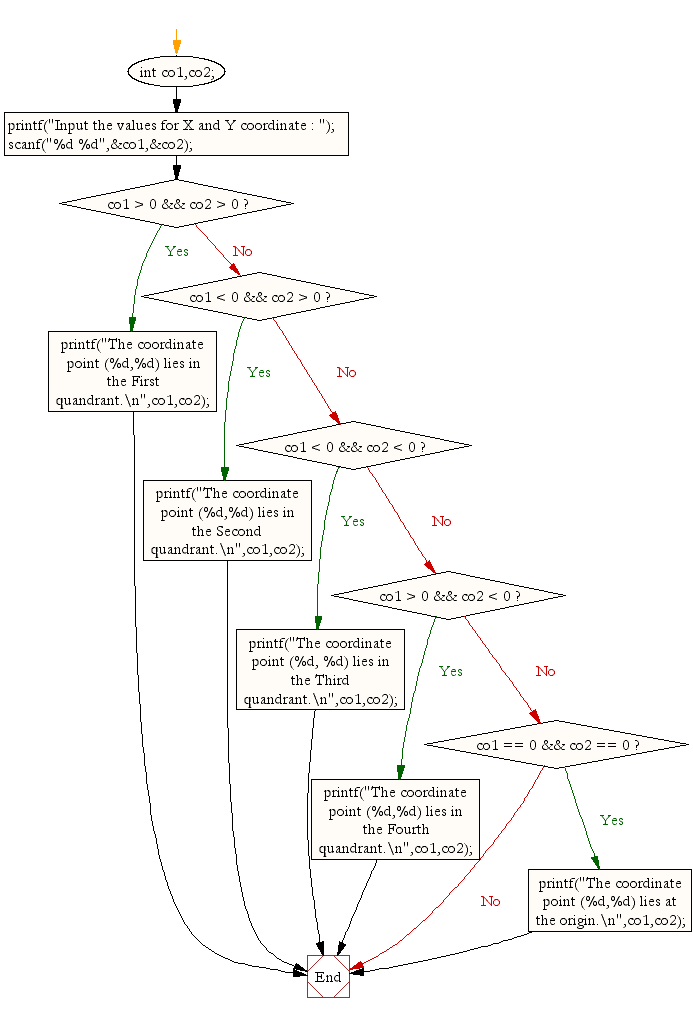﻿ C : Find the quadrant in which the coordinate point lies# C Exercises: Find the quadrant in which the coordinate point lies

## C Conditional Statement: Exercise-9 with Solution

Write a C program to accept a coordinate point in a XY coordinate system and determine in which quadrant the coordinate point lies.

Pictorial Presentation:Sample Solution:

C Code:

``````#include <stdio.h>
void main()
{
int co1,co2;

printf("Input the values for X and Y coordinate : ");
scanf("%d %d",&co1,&co2);

if( co1 > 0 && co2 > 0)
printf("The coordinate point (%d,%d) lies in the First quandrant.\n",co1,co2);
else if( co1 < 0 && co2 > 0)
printf("The coordinate point (%d,%d) lies in the Second quandrant.\n",co1,co2);
else if( co1 < 0 && co2 < 0)
printf("The coordinate point (%d, %d) lies in the Third quandrant.\n",co1,co2);
else if( co1 > 0 && co2 < 0)
printf("The coordinate point (%d,%d) lies in the Fourth quandrant.\n",co1,co2);
else if( co1 == 0 && co2 == 0)
printf("The coordinate point (%d,%d) lies at the origin.\n",co1,co2);

}
```
```

Sample Output:

```Input the values for X and Y coordinate : 7 9
The coordinate point (7,9) lies in the First quandrant.
```

Flowchart:C Programming Code Editor:

Improve this sample solution and post your code through Disqus.

What is the difficulty level of this exercise?

Test your Programming skills with w3resource's quiz.

﻿

## C Programming: Tips of the Day

Why do C and C++ compilers allow array lengths in function signatures when they're never enforced?

It is a quirk of the syntax for passing arrays to functions.

Actually it is not possible to pass an array in C. If you write syntax that looks like it should pass the array, what actually happens is that a pointer to the first element of the array is passed instead.

Since the pointer does not include any length information, the contents of your [] in the function formal parameter list are actually ignored.

Ref : https://bit.ly/3fhlvdH